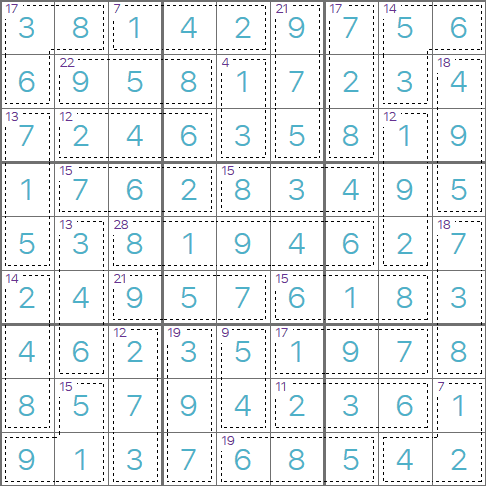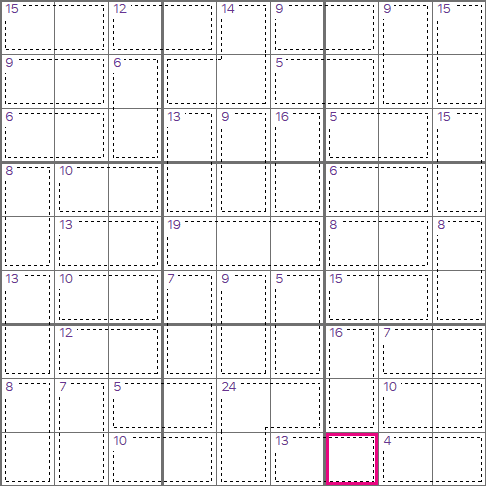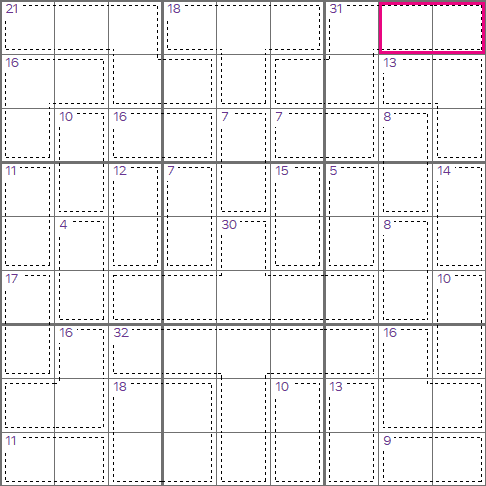# Killer Sudoku Rules

### A brief guideThis is an example of a completed Killer Sudoku puzzle. The grid is split up in to cages; each cage contains 2 or more cells, and has a total in the top-left corner. The cells in each cage must add up to the given total.
As an example, the cage in the top-left of this puzzle contains three cells, and they must add up to 17. We can check, and 3+8+6 does give 17.
Killer Sudoku puzzle are much harder than normal Sudoku puzzles, you should be able to comfortably solve a difficult Sudoku puzzle before trying to solve a Killer Sudoku puzzle.This is a Killer Sudoku starting grid, the first thing you'll need notice is that there are no starting numbers! We need to use a different set of techniques to get started with Killer Sudoku puzzles. We know that each row column and 3x3 region will contain the numbers 1-9, if we add these together we get 1+2+3+4+5+6+7+8+9=45, i.e. each row, column, and 3x3 region must add to 45/
Look at the bottom-right 3x3 region, we have 4 cages that add to 16+7+10+4=37. These cages between them cover 8 out of the 9 cells in this region. That means the remaining (highlighted) cell must be 45-37=8.
This is a great technique to use when starting with Killer Sudoku grids. You will also use techniques that you might use for Mathdoku puzzles. For example, the cage with 2 cells adding to 4 in the bottom-right only has one combination, i.e. 1+3=4. We can pencil these in and use the naked pairs technique.This is another example Killer Sudoku starting grid. We can't use the same technique for this grid, but we can use something very similar. Consider the two right-most columns, we know these cells must add to 90, i.e. 2x45. All but two of the cells (the highlighted ones) in these two columns are contained in a set of cages, and these cages don't cross in to the adjacent column. Adding these cage totals up gives 13+8+14+8+10+16+9=78. That means the two highlighted cells must add up to 90-78=12, this gives us a little more information about those cells than we had previously!